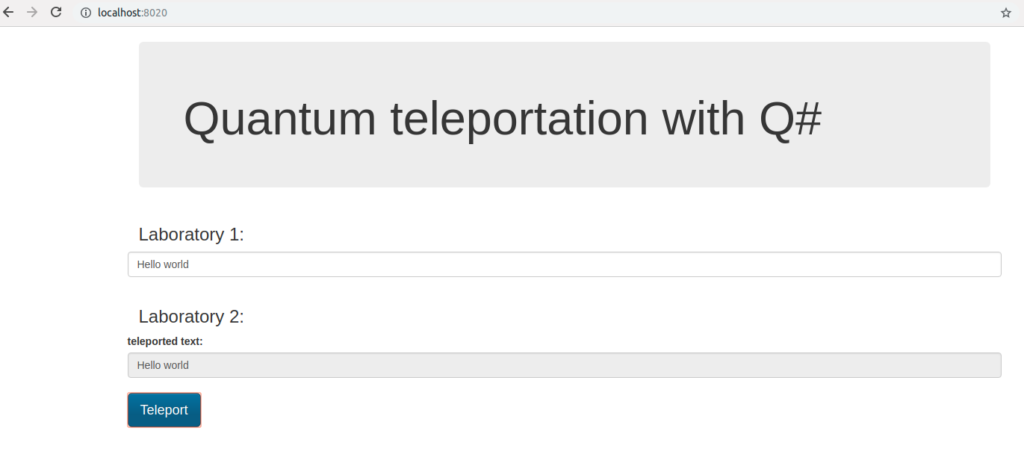# Quantum teleportation do it yourself with Q#Quantum computing nowadays is the one of the hottest topics in the computer science world. Recently IBM unveiled the IBM Q System One: a 20-qubit quantum computer which is touting as “the world’s first fully integrated universal quantum computing system designed for scientific and commercial use”.

In this article I’d like how to show the quantum teleportation phenomenon. I will use the Q# language designed by Microsoft to simplify creating quantum algorithms.

In this example I have used the quantum simulator which I have wrapped with the REST api and put into the docker image.

Quantum teleportation allows moving a quantum state from one location to another. Shared quantum entanglement between two particles in the sending and receiving locations is used to do this without having to move physical particles along with it.

# 1. Theory

Let’s assume that we want to send the message, specific quantum state described using Dirac notation:

 [latex display=”true”] \psi\rangle=\alpha 0\rangle+\beta 1\rangle[/latex]

Additionally we have two entangled qubits, first in Laboratory 1 and second in Laboratory 2:

 [latex display=”true”] \phi^+\rangle=\frac{1}{\sqrt{2}}( 00\rangle+ 11\rangle)[/latex]

thus we starting with the input state:

 [latex display=”true”] \psi\rangle \phi^+\rangle=(\alpha 0\rangle+\beta 1\rangle)(\frac{1}{\sqrt{2}}( 00\rangle+ 11\rangle))[/latex]
 [latex display=”true”] \psi\rangle \phi^+\rangle=\frac{\alpha}{\sqrt{2}} 000\rangle + \frac{\alpha}{\sqrt{2}} 011\rangle + \frac{\beta}{\sqrt{2}} 100\rangle + \frac{\beta}{\sqrt{2}} 111\rangle [/latex]

To send the message we need to start with two operations applying CNOT and then Hadamard gate.

CNOT gate flips the second qubit only if the first qubit is 1.

Applying CNOT gate will modify the first qubit of the input state and will result in:

 [latex display=”true”]\frac{\alpha}{\sqrt{2}} 000\rangle + \frac{\alpha}{\sqrt{2}} 011\rangle + \frac{\beta}{\sqrt{2}} 110\rangle + \frac{\beta}{\sqrt{2}} 101\rangle[/latex]

Hadamard gate changes states as follows:

 [latex display=”true”] 0\rangle \rightarrow \frac{1}{\sqrt{2}}( 0\rangle+ 1\rangle))[/latex]

and

 [latex display=”true”] 1\rangle \rightarrow \frac{1}{\sqrt{2}}( 0\rangle- 1\rangle))[/latex]

 [latex display=”true”]\frac{\alpha}{\sqrt{2}}(\frac{1}{\sqrt{2}}( 0\rangle+ 1\rangle)) 00\rangle + \frac{\alpha}{\sqrt{2}}(\frac{1}{\sqrt{2}}( 0\rangle+ 1\rangle)) 11\rangle + \frac{\beta}{\sqrt{2}}(\frac{1}{\sqrt{2}}( 0\rangle- 1\rangle)) 10\rangle + \frac{\beta}{\sqrt{2}}(\frac{1}{\sqrt{2}}( 0\rangle- 1\rangle)) 01\rangle[/latex]

and:

 [latex display=”true”]\frac{1}{2}(\alpha 000\rangle+\alpha 100\rangle+\alpha 011\rangle+\alpha 111\rangle+\beta 010\rangle-\beta 110\rangle+\beta 001\rangle-\beta 101\rangle)[/latex]

which we can write as:

 [latex display=”true”]\frac{1}{2}( 00\rangle(\alpha 0\rangle+\beta 1\rangle)+ 01\rangle(\alpha 1\rangle+\beta 0\rangle)+ 10\rangle(\alpha 0\rangle-\beta 1\rangle)+ 11\rangle(\alpha 1\rangle-\beta 0\rangle))[/latex]

Then we measure the states of the first two qubits (message qubit and Laboratory 1 qubit) where we can have four results:

•  $00\rangle$ which simplifies equation to: $00\rangle(\alpha 0\rangle+\beta 1\rangle)$ and indicates that the qubit in the Laboratory 2 is $\alpha 0\rangle+\beta 1\rangle$
•  $01\rangle$ which simplifies equation to: $01\rangle(\alpha 1\rangle+\beta 0\rangle)$ and indicates that the qubit in the Laboratory 2 is $\alpha 1\rangle+\beta 0\rangle$
•  $10\rangle$ which simplifies equation to: $10\rangle(\alpha 0\rangle-\beta 1\rangle)$ and indicates that the qubit in the Laboratory 2 is $\alpha 0\rangle-\beta 1\rangle$
•  $11\rangle$ which simplifies equation to: $11\rangle(\alpha 1\rangle-\beta 0\rangle)$ and indicates that the qubit in the Laboratory 2 is $\alpha 1\rangle-\beta 0\rangle$

Now we have to send the result classical way from Laboratory 1 to Laboratory 2.

Finally we know what transformation we need to apply to qubit in the Laboratory 2 to make its state equal to message qubit:

 [latex display=”true”] \psi\rangle=\alpha 0\rangle+\beta 1\rangle[/latex]

if Laboratory 2 qubit is in state:

•  $\alpha 0\rangle+\beta 1\rangle$ we don’t need to do anything.
•  $\alpha 1\rangle+\beta 0\rangle$ we need to apply NOT gate.
•  $\alpha 0\rangle-\beta 1\rangle$ we need to apply Z gate.
•  $\alpha 1\rangle-\beta 0\rangle$ we need to apply NOT gate followed by Z gate

This operations will transform Laboratory 2 qubit state to initial message qubit state thus we moved the particle state from Laboratory 1 to Laboratory 2 without moving particle.

# 2. Code

Now it’s time to show the quantum teleportation using Q# language. I have used Microsoft Quantum Development Kit to run the Q# code inside the .NET Core application. Additionally I have added the nginx proxy with the angular gui which will help to show the results. Everything was put inside the docker to simplify the setup.

Before you will start you will need git, docker and docker-compose installed on your machine (https://docs.docker.com/get-started/)

To run the project we have to clone the repository and run it using docker compose:

git clone https://github.com/qooba/quantum-teleportation-qsharp.git
cd quantum-teleportation-qsharp
docker-compose -f app/docker-compose.yml up


Now we can run the http://localhost:8020/ in the browser:Then we can put the message in the Laboratory 1, click the Teleport button, trigger for the teleportation process which sends the message to the Laboratory 2.

The text is converted into array of bits and each bit is sent to the Laboratory 2 using quantum teleportation.

In the first step we encode the incoming message using X gate.

if (message) {
X(msg);
}


Then we prepare the entanglement between the qubits in the Laboratory 1 and Laboratory 2.

H(here);
CNOT(here, there);


In the second step we apply CNOT and Hadamard gate to send the message:

CNOT(msg, here);
H(msg);


Finally we measure the message qubit and the Laboratory 1 qubit:

if (M(msg) == One) {
Z(there);
}

if (M(here) == One) {
X(there);
}

 If the message qubit has state $1\rangle$ then we need to apply the Z gate to the Laboratory 2 qubit. If the Laboratory 1 qubit has state $1\rangle$ then we need to apply the X gate to the Laboratory 2 qubit. This information must be sent classical way to the Laboratory 2.

Now the Laboratory 2 qubit state is equal to the initial message qubit state and we can check it:

if (M(there) == One) {
set measurement = true;
}


This kind of communication is secure because even if someone will take over the information sent classical way it is still impossible to decode the message.